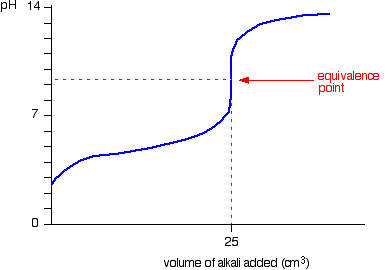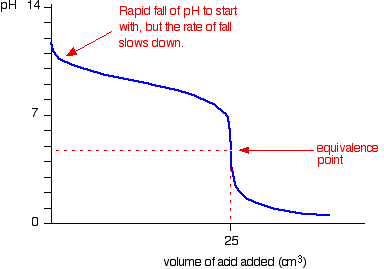# 18.3 pH curves

### Buffer solutions

• A buffer is a solution that resists a change in pH upon addition of small amounts of a strong base, strong acid or water.
• Buffer Is composed of weak acid and its conjugate base, or a weak base and its conjugate acid

### pH curves

• There are 4 possibilites of pH curves
• Strong acid – Strong base
• Starting point on pH axis is important as it gives initial pH of acid• Weak acid – Strong base
• Starting point on pH axis is important as it gives initial pH of acid
• Half equivalence point is the stage at which half the amount of weak acid has been neutralized
• At this point, pKa=pH• Strong acid – Weak base
• Starting point on pH axis is important as it gives initial pH of base
• Half equivalence point is the stage at which half the amount of weak base has been neutralized
• At this point, pKb = pOH• Weak acid – Weak base
• Starting point on pH axis is important as it gives initial pH of base
• Gradual change, equivalence point is hard to determine, graph has no purpose